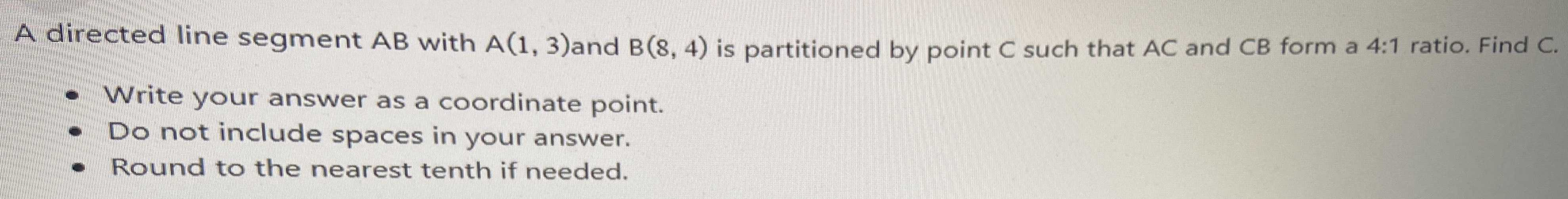### ¿Todavía tienes preguntas de matemáticas?

Pregunte a nuestros tutores expertos
Algebra
PreguntaA directed line segment $$A B$$ with $$A ( 1,3 )$$ and $$B ( 8,4 )$$ is partitioned by point $$C$$ such that $$A C$$ and $$C B$$ form a $$4 : 1$$ ratio. Find $$C$$ .